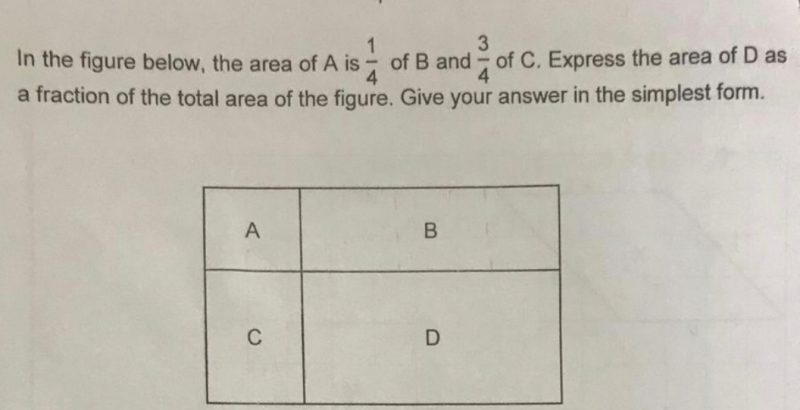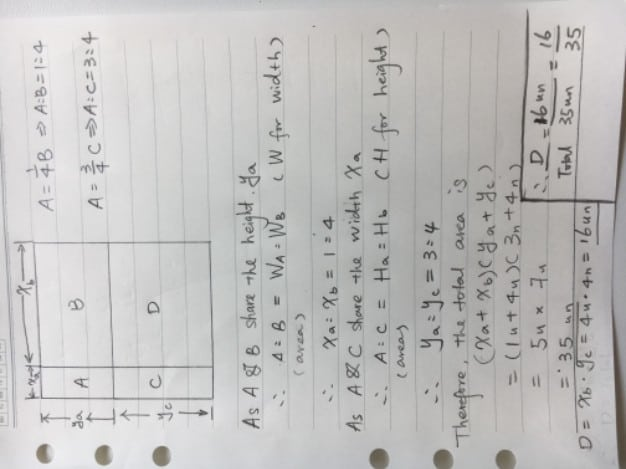Question**Dear Teachers, please help to solve this question. Thank you.

A : B
1 : 4

A : C
3 : 4

A : B : C
3 : 12 : 4

12/3 = 4
4 x 4 = 16 (area of D)
16/(3 + 12 + 4 + 16) = 16/35

Ans : 16/35.

0 Replies 0 Likes

Let A = 3,

so, B is 12, as A is 1/4 of B;

and C is 4, as A is 3/4 of C;

and D is 16, as D is 4 times of C;

total area is 3+4+12+16=35

so D to overall area is 16/35

0 Replies 1 Like0 Replies 0 Likes